SCHOLASTIC APTITUDE TEST 1997.

PHYSICS

 Time: ONE Hour Max. Marks: 60 (10.45 AM - 11.45 AM)

• Answers must be written either in English or the medium of instruction of the candidate in high school.
• There will be no negative marking.
• There are THREE sections. Answer all questions of a section at one place.
• The relevant working in arriving at an answer has to be shown wherever required. However if the answer can be arrived at without detailed working, write only the answer
• Use of calculators or graph papers is not permitted.

Marks allotted for individual sections are given below. All questions in an individual section carry equal marks.

Section A      10 Marks
Section B      20 Marks
Section C      30 Marks

Useful Physical constants:

1. acceleration due to gravity = 10 ms–2
2. Charge of electron e = 1.6 x 10-19 C
3. 1/4p e o = 9 x 109 Nm2C–2
4. Density of wood = 0.5 gm/cc

SECTION A

1. A wire carries a current vertically downwards. The magnetic field to the East of the wire is directed towards                               .
2. A source of sound is moving at 36 kmph straight towards an observer moving away from it at 36 kmph and along the same straight line. The frequency of the source is 400 Hz. Frequency of the note received by the observer is                           .
3. If 0.16 mA of current is flowing through a wire, the number of electrons crossing any section of the wire in a minute is                               .
4. A hypothetical radioactive nucleus emits a proton and a positron. It’s mass number decreases by                           and it’s charge number decreases by                         .
5. Mass equivalent of energy of 186.2 MeV is                                   amu.
6. In electrolysis of copper sulphate solution, an alternating current source is used. The mass of copper deposited on the cathode plate is                            .
7. In wavelength of a wave in a certain medium is 20 cm and its velocity is 350 m/s. Its wavelength in a medium where the velocity is 1400 m/s would be equal to                                .
8. The energy consumed by a 20 W lamp used for 25 hrs is                      kWh
9. If a cell of emf 6 V drives a current of 2 amp through a circuit for 10 minutes, the amount of work done by it is                              .
10. 20 mJ of work is done in moving one micro Coloumb of charge between two points. The potential difference between these points is                                   .

SECTION B

1. Light travels at v1 in a medium and at v2 in another medium. The ratio of refractive indices of the media is                               .
2. The closest distance between particles one at an extreme position and another at the equilibrium position is 2 m. If the frequency of this wave is 2.5 kHz, the velocity of the wave is                              .
3. At 1 atmosphere pressure and a certain temperature the velocity of sound is 350 m/s. At 2 atmosphere pressure and same temperature the velocity would be                                   .
4. Two stones are released from rest one from a height of 9 m and another from some unknown height above the ground. One of the stones hits the ground 1 s after the other strikes the ground. The height from which the second stone is released can have values of                              .
5. Light falling on a sphere made of a photoelectric material causes it to lose electrons. If a sphere initially uncharged has acquired a charge of 3.2 m C, the number of electrons it has lost is                                   .
6. Energy stored by a capacitor is given by the expression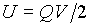. If a 5 m F capacitor charged to 200 V, the energy it possesses is equal to                            .
7. 2 kgf/cm2 =                              N/m2.( kgf = g N )
8. Momentum is mv and kinetic energy is mv2/2. A body of mass 4 kg has a kinetic energy of 32 J. It’s momentum is                       .
9. A solid object A of mass 200 gm object placed in contact with a source supplying heat at a constant rate undergoes a rise in temperature of 20 C° in 40 s. Another solid object B of mass 300 gm needs 20 s for a 5 C° rise in temperature. The ratio of specific heats of A and B is                                     .
10. Mass of a radio active substance at a certain moment is 16 gm. The mass of it that disintegrates in it’s third half-life period is                       .

SECTION C

In some of the following questions information ( A new rule, a new equation…) required to answer the questions is provided. Read the information provided carefully and answer the following question. The questions can be answered by proper appreciation of the information provided.

 Resultant R of two parallel forces P and Q acting at A and B is equal to P+Q and the point C where it acts can be located from the relation P(AC)=Q(BC). ( Refer to the figure shown below )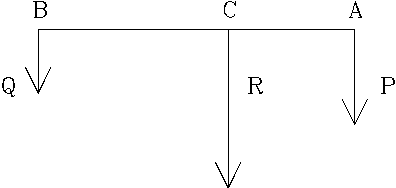The resultant of two parallel forces is 40 N and acts 10 cm from the force of 10 N. The other force acts at a distance of                                from the 10 N force.
1. A stone projected in to the air from the ground level with a velocity v directed at q above the horizontal hits the ground at a horizontal distance of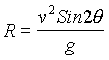from the point of projection. The maximum height attained by it during the flight is given by the expression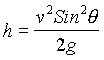.

A projectile hurled at 45° attains a maximum height of 25 m. The horizontal distance it travels before it strikes the ground is                                     .

1. A positively charged particle travelling vertically upwards experiences a northward force due to magnetic field directed at right angles to it. A negatively charged particle travelling northward in the same magnetic field will experience a                         force.
1. A long uniform wooden stick of cylindrical cross section is weighted at the bottom such that a length of 20 cm of it protrudes out of water as it floats vertically. If a length of 10 cm is sawed off from the stick and it is floated again, the length that protrudes above the water surface would be                    .
2. A lens forms a twice magnified image of an object kept 30 cm away from it on oneside on a screen kept on the other side. Without disturbing the object, the lens is moved through 5 cm towards the object, the screen must be moved by                                  to obtain a sharp image.
 Between any two adjacent junctions the resistance of a wire frame shown is same. If the resistance between B and E is R, resistance between A and B is                                          .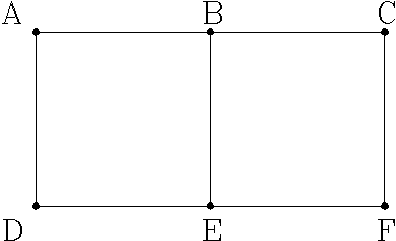1. An insect is located at the edge of a fan blade of length 40 cm. It starts walking toward the centre of the fan. As the insect moves 10 cm from its initial position, the blade has turns through a right angle. The distance between initial and final positions is                                     .
2. Two cars are travelling towards a junction along two different intersecting roads. One of them is 200 m away and is moving uniformly at 10 m/s. The other located 100 m away starts from rest and accelerates uniformly at 2 m/s2 towards the junction. The interval between the moments when the cars pass the intersection is                              .
3. A wire is of square cross section of side ‘a’ and length L and has a resistance R. Another wire made of same material and of twice greater length has the same volume as the first. The electrical resistance of the second wire is                       .
4. A ring with a uniformly distributed charge (Q) of radius R repels a point charge (q) placed on its axis with a force F given by the expression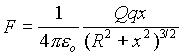where x is the distance of q from the centre of the ring.

A point charge of 5 m C is located on the axis of a ring of radius 60 cm carrying a charge of 40 m C. The distance between any point on the ring and the point charge is 100 cm. The force experienced by the point charge is                                   .